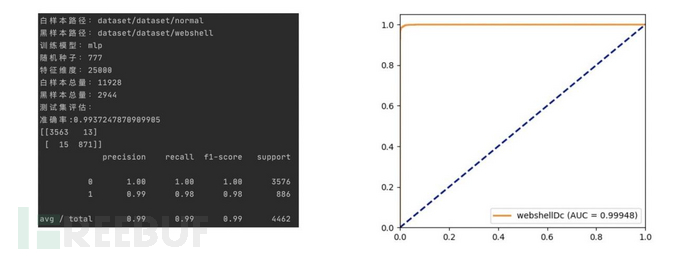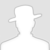FreeBuf.COM网络安全行业门户，每日发布专业的安全资讯、技术剖析。FreeBuf+小程序

2020-11-16 16:02:46

# WebShell脚本检测机器学习实践

webshell作为黑客惯用的入侵工具，是以php、asp、jsp、perl、cgi、py等网页文件形式存在的一种命令执行环境。黑客在入侵一个网站服务器后，通常会将webshell后门文件与网站服务器WEB目录下正常网页文件混在一起，通过Web访问webshell后门进行文件上传下载、访问数据库、系统命令调用等各种高危操作，达到非法控制网站服务器的目的，具备威胁程度高，隐蔽性极强等特点。

## 1 静态检测思路

vps提权马

asp一句话

backdoor.php

### 1.2 特征码匹配法

```rule=r'(array_map[\s\n]{0,20}\(.{1,5}(eval|assert|ass\\x65rt).{1,20}\\$_(GET|POST|REQUEST).{0,15})'
rule='(call_user_func[\s\n]{0,25}\(.{0,25}\\$_(GET|POST|REQUEST).{0,15})'
rule='(\\$_(GET|POST|REQUEST)\[.{0,15}\]\s{0,10}\(\s{0,10}\\$_(GET|POST|REQUEST).{0,15})'
rule='((\\$(_(GET|POST|REQUEST|SESSION|SERVER)(\[[\'"]{0,1})\w{1,12}([\'"]{0,1}\])|\w{1,10}))[\s\n]{0,20}\([\s\n]{0,20}(@{0,1}\\$(_(GET|POST|REQUEST|SESSION|SERVER)(\[[\'"]{0,1})\w{1,12}([\'"]{0,1}\])|\w{1,10}))[\s\n]{0,5}\))'
rule='\s{0,10}=\s{0,10}[{@]{0,2}(\\$_(GET|POST|REQUEST)|file_get_contents|str_replace|["\']a["\']\.["\']s["\']\.|["\']e["\']\.["\']v["\']\.|["\']ass["\']\.).{0,10}'
rule='((eval|assert)[\s|\n]{0,30}\([\s|\n]{0,30}(\\\\{0,1}\\$((_(GET|POST|REQUEST|SESSION|SERVER)(\[[\'"]{0,1})[\w\(\)]{0,15}([\'"]{0,1}\]))|\w{1,10}))\s{0,5}\))'
rule='((eval|assert)[\s|\n]{0,30}\((gzuncompress|gzinflate\(){0,1}[\s|\n]{0,30}base64_decode.{0,100})'
rule='\s{0,10}=\s{0,10}([{@]{0,2}\\\\{0,1}\\$_(GET|POST|REQUEST)|file_get_contents|["\']a["\']\.["\']s["\']\.|["\']e["\']\.["\']v["\']\.|["\']ass["\']\.).{0,20}'
rule='(include|require)(_once){0,1}[\s*]+[\"|\']+[0-9A-Za-z_]*\://'
rule='([^\'"](include|require)(_once){0,1}\s{0,5}(\s{0,5}|\(\s{0,5})["\']([\.\w\,/\\\+-_]{1,60})["\']\s*\){0,1})'
rule='((include|require)(_once){0,1}(\s{0,5}|\s{0,5}\(\s{0,5})[\'"]{0,1}(\\$(_(GET|POST|REQUEST|SERVER)(\[[\'"]{0,1})\w{0,8}([\'"]{0,1}\])|[\w]{1,15}))[\'"]{0,1})'
rule='\s{0,10}=\s{0,10}([{@]{0,2}\\$_(GET|POST|REQUEST)|[\'"]{0,1}php://input[\'"]{0,1}|file_get_contents).{0,20}'
rule='gzdeflate|gzcompress|gzencode'
rule = '(preg_replace[\s\n]{0,10}\([\s\n]{0,10}((["\'].{0,15}[/@\'][is]{0,2}e[is]{0,2}["\'])|\\$[a-zA-Z_][\w"\'\[\]]{0,15})\s{0,5},\s{0,5}.{0,40}(\\$_(GET|POST|REQUEST|SESSION|SERVER)|str_rot13|urldecode).{0,30})' ```

### 1.3 语义分析

Pecker Scanner检测工具就是基于语法的php文件webshell检测方法。该方法对检测文件代码进行清洗后，分析其变量、函数、字符串来实现关键危险函数的捕获，这样可以很好地解决漏报，但同时也存在大量误报。

### 1.4 统计特征检测

1. 字符级重合指数(LanguageIC): 字符重合指数越低，说明代码越混乱，有可能被加密或混淆过
2. 字符级信息墒（Entropy）：度量代码中所使用字符的不确定性，可用gini系数来代替使计算更高效，二者具备相似性质（跟上面的字符重合指数也具有相似的效果）
3. 最长单词长度(LongestWord)：最长的字符串一般是base64编码，存在被编码或被混淆的可能
4. 恶意代码签名特征(SignatureNasty)：统计代码中包含的恶意代码片段个数
5. 压缩特征(Compression)：计算代码文件压缩比

## 2 机器学习方法实践

### 2.2 特征处理和模型训练

```def features_process(negativedir, postivedir, maxfeatures):
webshell_number = len(webshell_texts)
normal_number = len(normal_texts)
texts = webshell_texts + normal_texts
webshell_lables =  * webshell_number
normal_lables =  * normal_number
lables = webshell_lables + normal_lables
logger.info("白样本总量：%i" % normal_number)
logger.info("黑样本总量：%i" % webshell_number)

countvectorizer = CountVectorizer(ngram_range=(2, 2), decode_error="ignore",
min_df=1, analyzer="word",
token_pattern=r'[^\w\s]+|\b\w+\b',
max_features=maxfeatures)
tfidftransformer = TfidfTransformer(smooth_idf=False)
cv_x = countvectorizer.fit_transform(texts).toarray()
tf_x = tfidftransformer.fit_transform(cv_x).toarray()

joblib.dump(countvectorizer, "model/countvectorizer_" + options.version + ".pkl")
joblib.dump(tfidftransformer, "model/tfidftransformer_" + options.version + ".pkl")
return tf_x, lables, countvectorizer, tfidftransformer

def evaluation(y_test, y_pred):
logger.info("准确率:%s" % metrics.accuracy_score(y_test, y_pred))
logger.info(confusion_matrix(y_test, y_pred))
logger.info(classification_report(y_test, y_pred))

def train(trainset, lables, mode, seed):
x_train, x_test, y_train, y_test = train_test_split(trainset, lables, test_size=0.3, random_state=seed)
clf = model_collection(mode)
clfname = "model/" + mode + "_" + options.version + ".pkl"
clf.fit(x_train, y_train)
logger.info("训练集评估：")
evaluation(y_train, clf.predict(x_train))
logger.info("测试集评估：")
evaluation(y_test, clf.predict(x_test))
joblib.dump(clf, clfname, compress=3)```

### 2.3 评估## 3 结语

# webshell检测

+ 收入我的专辑• 0 文章数
• 0 评论数
• 0 关注者